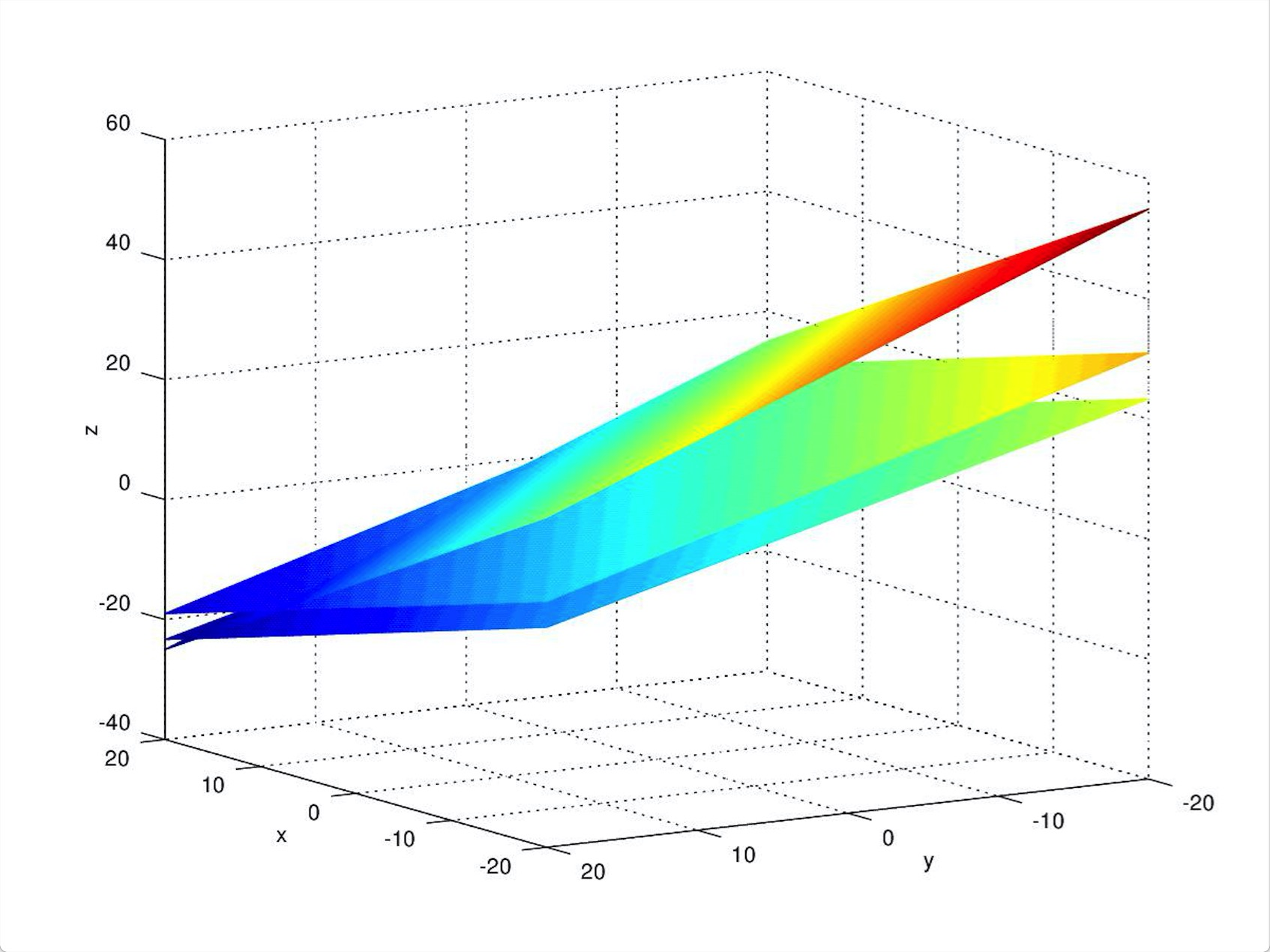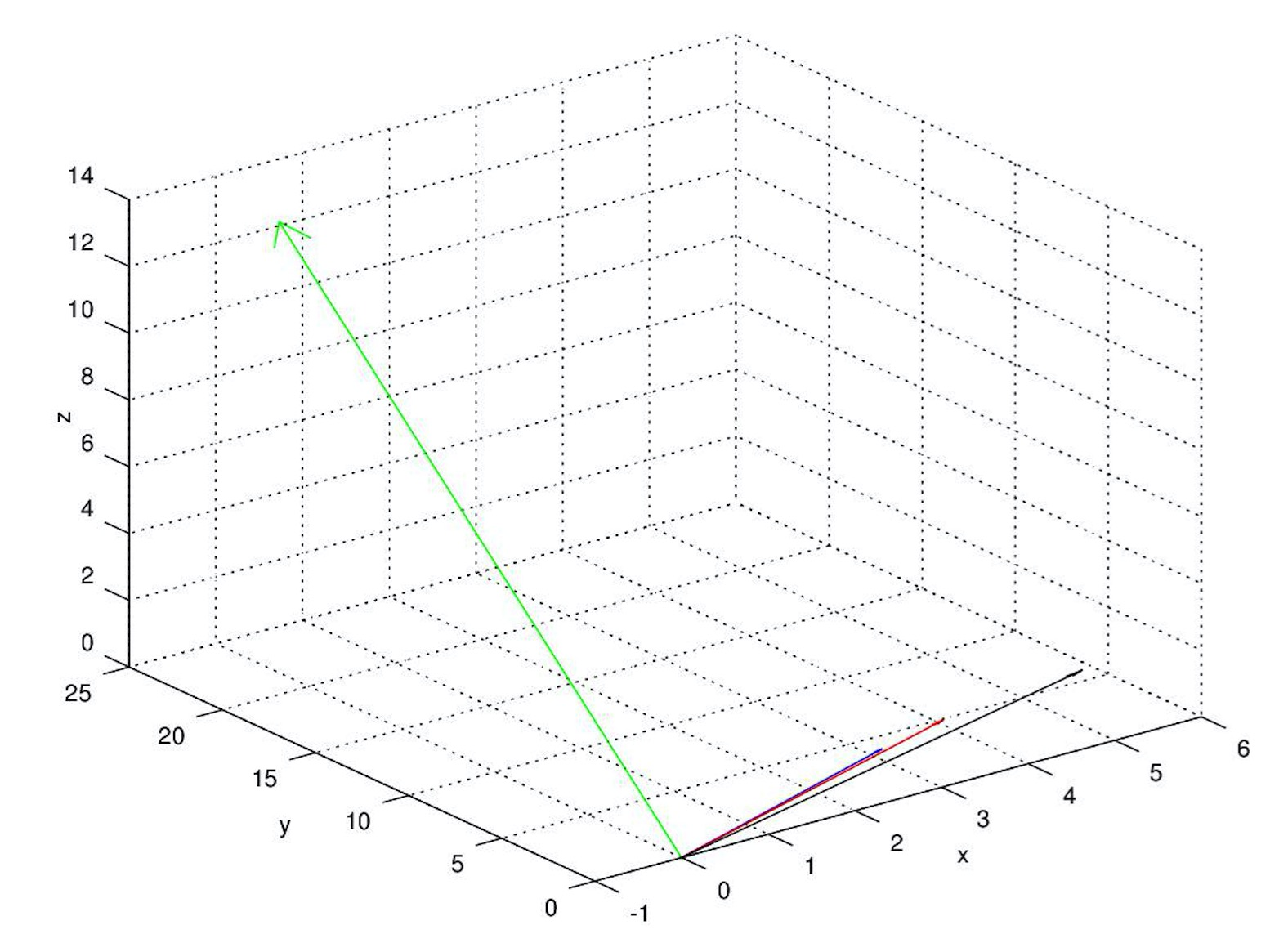### Motivation

When I was learning Linear Algebra in University, I found it was hard to understand what would applications of this course. I remember that we learned determinant on Chapter 1, then we learned a lot of other concept such as Rank and EigenValue. I could only memorize one after another theorems without knowing why these happened to appear this way. However, when I was learning matrices from lectures taught by Gilbert Strang, I found every conclusion is so nature that I could easily understand and associate a bunch of concepts with each other. I’d like to conclude what I had learned from him as well as hoping that someone can learn from that.

### Why this subject exists?

First of all, what is the origin of linear algebra? Why we make the world increasingly complicated? The truth is that the purpose of mathmatics is to make the world simpler, not the opposite, Linear Algebra as well. For example, we want to solve equations with a lot of variables, the original way is to write all the parameter out：
\begin{cases}
3x+4y+6z = 0\\
2x+3y+4z = 24\\
x+y+z = 15
\end{cases}

If there are only 3 parameters, the problems is not so hard for us to handle. But what if there are 100 parameters? When we transfer the equation to matrices, the outcome becomes so grateful:
\begin{align}
\begin{bmatrix}
3&4&6\\
2&3&4\\
1&1&1
\end{bmatrix}
\begin{bmatrix}
x\\
y\\
z
\end{bmatrix}=\begin{bmatrix}0\\24\\15\end{bmatrix}
\end{align}

### Solve the equation group

Now, we try to solve the problems, our instinction is to draw the three planes, under most circumstance, there will be a cross point, octave code and the plot is as followed:The answer of this equation group is that
\begin{align}
\begin{bmatrix}
x\\
y\\
z
\end{bmatrix}=\begin{bmatrix}
-18\\
72\\
-39
\end{bmatrix}
\end{align}

### Another perspective

We can find another perspective to solve this equation: see the equation as a combination of columns, that means:
\begin{align}
x*\begin{bmatrix}
3\\
2\\
1
\end{bmatrix}+y*
\begin{bmatrix}
4\\
3\\
1
\end{bmatrix}+z*
\begin{bmatrix}
6\\
4\\
1
\end{bmatrix}=
\begin{bmatrix}
0\\
24\\
15
\end{bmatrix}
\end{align}
Now, we can acquire the new vector through three vectors’ combination, from the plot blow demonstrates that we need to find a combination of red&blue&black vectors to generate another vector(the green one). Obviously, $x=-18,y=72,z=-39$ is the only solution of the combination problem.How to solve equations using program, and is there always an answer of equations? An easy way is to do elimination of these there equations. What now I want to do right now is to change these equation as follows:
\begin{cases}
3x+4y+6z = 0\\
(1/3)y+0z = 24\\
-z = 39
\end{cases}
Step1, calclate z, step2, calclate y, step3, calclate x. We first leave a variables z alone with an answer in the third equation. Then, we substitute z to the second equation, and get y. At last we substitute x and y to the first equation and get x. if we represent above through matrices language, it is like this:
\begin{align}
\begin{bmatrix}
3&4&6\\
0&1/3&0\\
0&0&-1
\end{bmatrix}
\begin{bmatrix}
x\\
y\\
z
\end{bmatrix}=
\begin{bmatrix}
0\\
24\\
39
\end{bmatrix}
\end{align}
The triangle at bottom-left side is all zeros which ensure we can solve equations one by one. The process is named back-substitution.

But what if the equation group looks like:
\begin{align}
\begin{bmatrix}
1&2&3\\
1&2&3\\
1&2&3
\end{bmatrix}
\begin{bmatrix}
x\\
y\\
z
\end{bmatrix}=
\begin{bmatrix}
5\\
6\\
7
\end{bmatrix}
or
\begin{bmatrix}
3&4\\
4&5\\
5&6\\
6&7\\
7&9
\end{bmatrix}
\begin{bmatrix}
x\\
y
\end{bmatrix}=
\begin{bmatrix}
5\\
5\\
5\\
5\\
5
\end{bmatrix}
\end{align}
Next time we will talk about LU decomposition and Rank of a matrix.